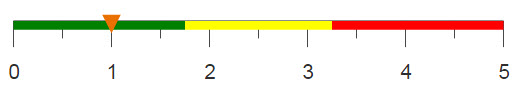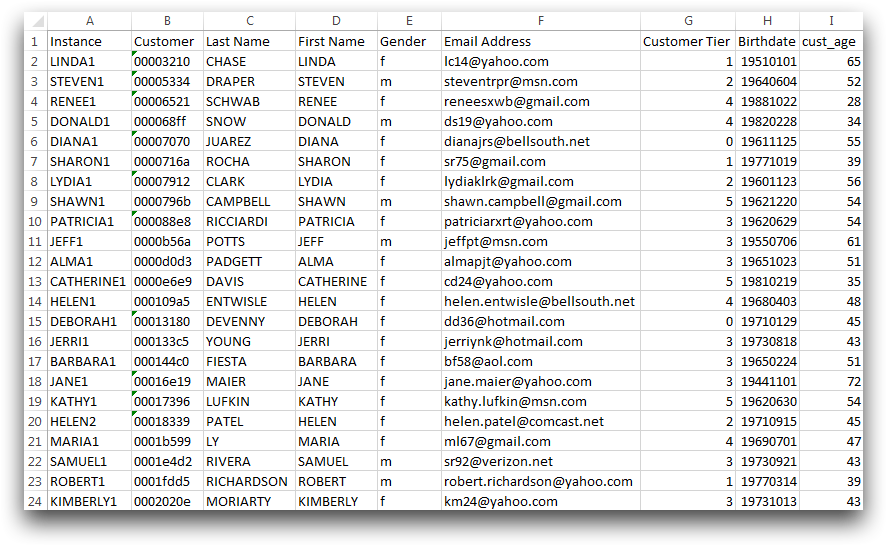# Finding a value corresponding to the nth element

Using the g_cumcnt function, you can find corresponding information related to a specific occurrence of a value, similar to using COUNTIF and VLOOKUP in Excel.

## Difficulty## Objective

You want to find out information for the specific occurrence of a value in your data table. For example, a store has a list of customers and you want to know the customer number of the 5th Aaron on the list. The VLOOKUP function in Excel returns the information in a specified column for a given value. However, this function will only return the information for the first instance. By combining the use of COUNTIF and VLOOKUP you can find the information for a specific occurrence of the value. Using 1010data, you can use g_cumcnt and a selection statement to determine the same information.

## Excel solution

VLOOKUP finds the position of the specified value and returns the corresponding information in a different column. Since this function will only return the information for the first instance of the value, you need to create a new column using COUNTIF to distinguish between each occurrence.This new column is now used in the VLOOKUP function. To determine the customer number of the 5th Aaron in the list you type the following equation in Excel:

`=VLOOKUP("AARON5",A:I,5,FALSE)`

This equation returns 0375421a.

Although this set of operations finds the desired information, it does not do so in an efficient manner. It should be noted that in order to use the VLOOKUP function to find a value, the column that contains the value must be listed first in the column order. Additionally, after executing the COUNTIF formula on the first cell, you must then apply it to each additional row in the table. This can be done with a simple click of the mouse, but will take several minutes to actually complete. The same results can be found using 1010data's platform in a fraction of the time.

## 1010data Macro Language solution

```<base table="pub.doc.retail.altseg.customers"/>
<willbe name="occurrence"
value="splice(firstName,g_cumcnt(firstName;;);'')"/>
<sel value="occurrence='AARON5'"/>
<colord cols="customer"/>```

Similar to the Excel method, an additional column needs to be created to distinguish the occurrences of each name. This can be done using splice to combine the first name with it's occurrence in the table, found using g_cumcnt(G;S;O).

Once this column is created, you can simply select the value in the column that is equal to the first name combined with the desired occurrence. Using colord to only show the customer column gives a similar look to the output of the Excel function.

## Further reading

If you would like to learn more about the functions and operations discussed in this recipe, click on the links below:

g_cumcnt(G;S;O)